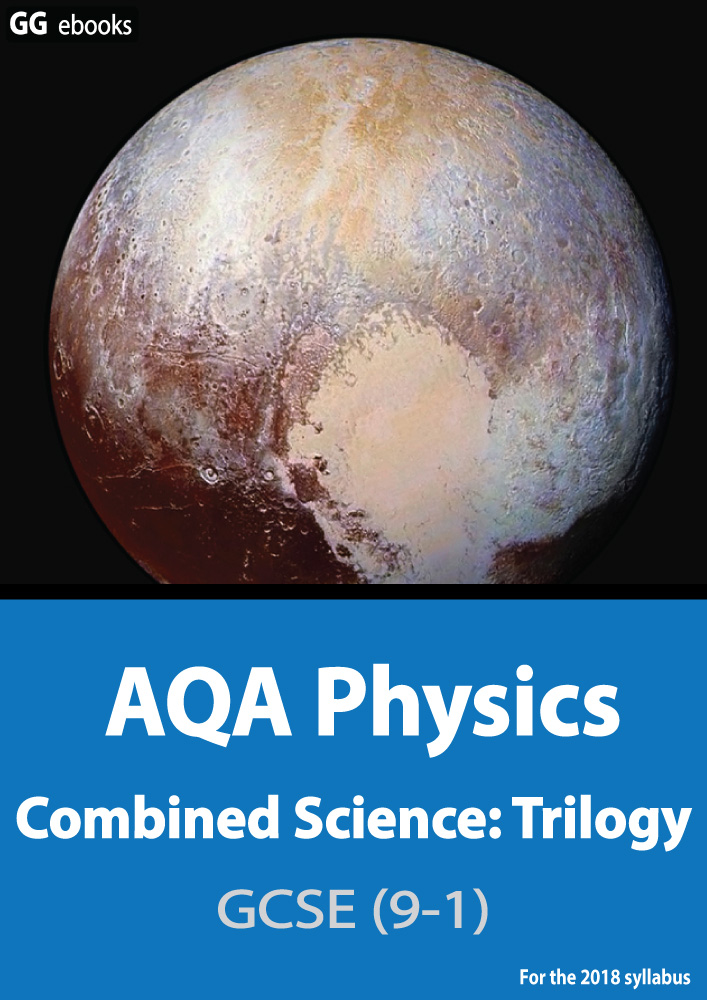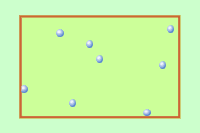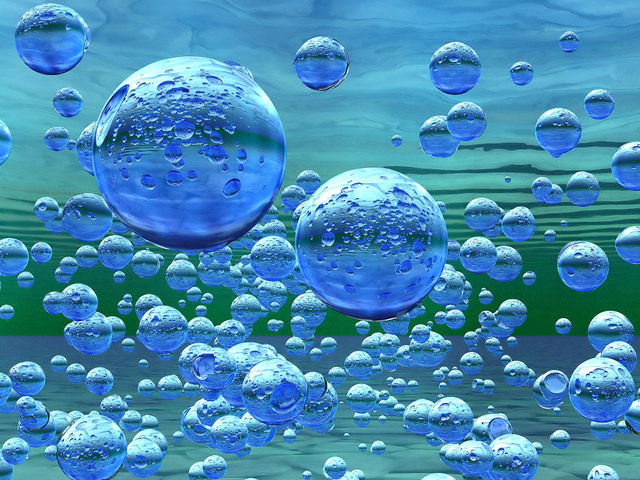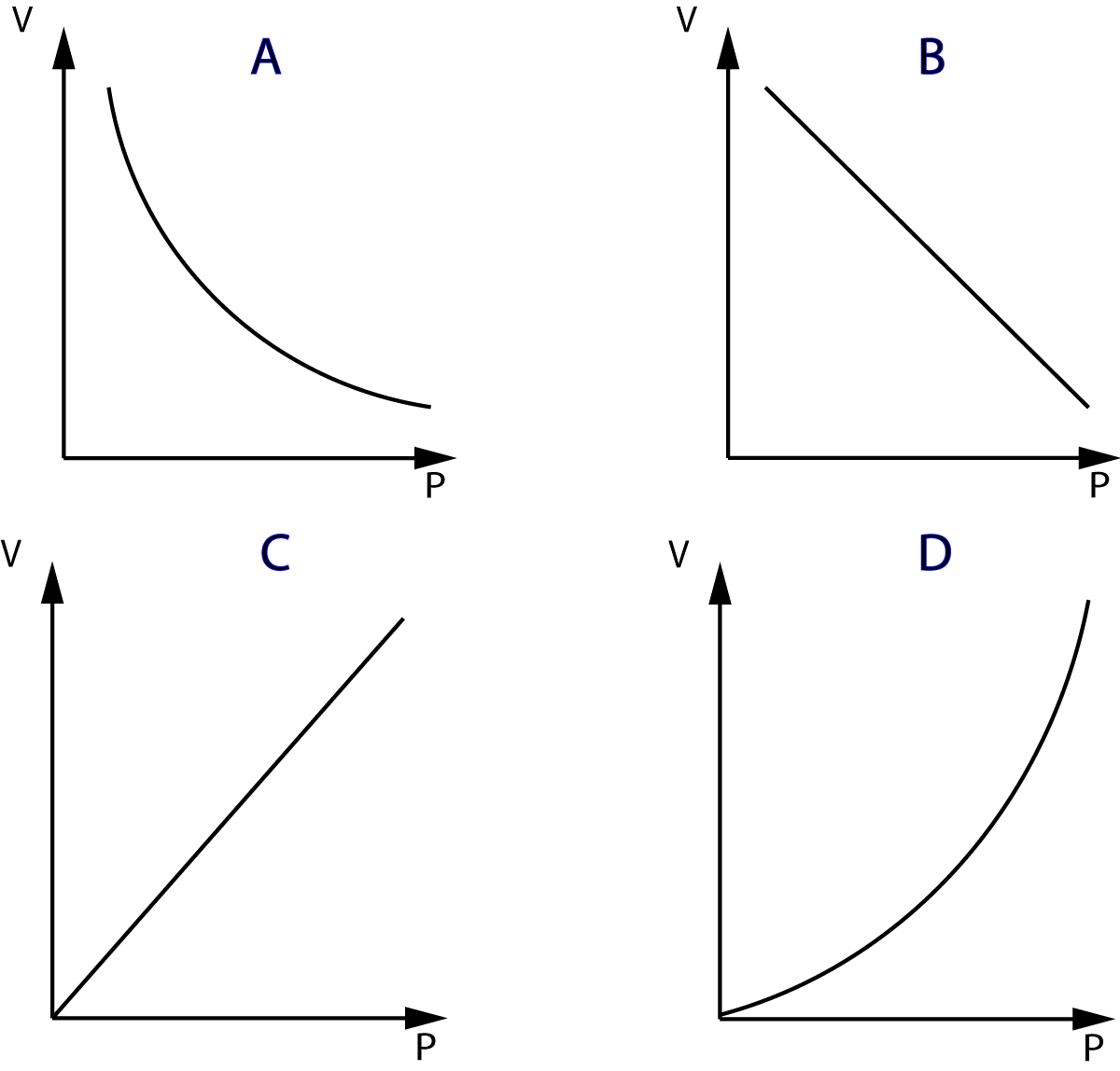NEED HELP? AQA GCSE digital textbooks From the makers of Grade Gorilla. GGebooks.com10 minutes maximum! Can you do it in 5?

Q1+2. The diagram shows gas molecules trapped in a container.

The molecules (particles) are moving very fast. they collide with the walls of the container producing a pressure.1. Which of these statements best describes the effect of this pressure on the container?

• A. The pressure produces a force parallel to the surface of the container.
• B. The pressure produces a force at right angles to the surface of the container.
• C. The pressure produces energy parallel to the surface of the container.
• D. The pressure produces energy at right angles to the surface of the container.

2. If the container volume is increased, without changing the temperature of the gas, what happens to pressure of the gas?

• A. The pressure increases because the particles move and collide faster.
• B. The pressure increases because the particles hit the sides of the container more frequently.
• C. The pressure decreases because the particles move and collide more slowly.
• D. The pressure decreases because the particles hit the sides of the container less frequently.

3-6. A simple experiment is carried out using a gas syringe:

A pressure sensor is attatched to the end of the syringe, and the pressure reading taken as the gas volume is reduced. The reading is taken after a while to allow the temperature to remain constant. The results are shown in the green table below.

The formula linking pressure and volume is:

p V = constantDatabase Center for Life Science (DBCLS)

3. What are the likely missing volume units labelled X in the results table below?

• A) cm2
• B) cm3
• C) m2
• D) m3

4-6. What are the missing values in the results table for the syringe shown here?

Volume
('X')

Pressure
(kPa)

6
100
120
3
2.5

7-10. The volume of an air bubble in water is 60cm3 at a pressure of 4 x 105 Pa. The bubble expands as it rises to the surface.

The formula linking pressure and volume is:    p V = constantH

7. Which of the following statements best describes the observations above?

• A) As the bubble's volume increases it makes the pressure increase.
• B) As the bubble's volume increases it makes the pressure decrease.
• C) As the pressure decreases, the bubble's volume increases.
• D) As the pressure decreases, the bubble's volume decreases.

8. What will be the volume of the bubble in cm3 at a pressure of 1 x 105 pascals?

• A) 15
• B) 40
• C) 60
• D) 240

9. During the bubble's ascent, what is the pressure of the water when the bubble has a volume of 80cm3?

• A) 2 x 105 Pa
• B) 3 x 105 Pa
• C) 4 x 105 Pa
• D) 5.33 x 105 Pa

10. Which of these graphs best shows the relationship between pressure and volume of a fixed mass of gas?